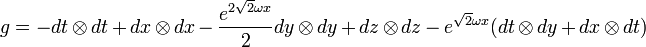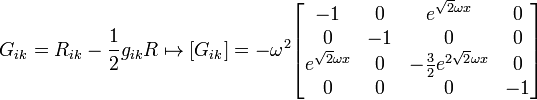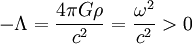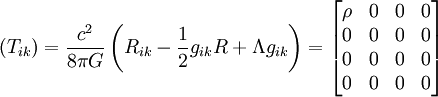## Metric form of Gödel's universe

The geometry of Gödel's universe is represented in a space-time where the metric can be represented by pseudo-Cartesian coordinates and units in which=1 in such a way:Whereis a constant, associated with the vorticity of the flow of matter, Furthermore, this vorticity can be related to the density of matter of this universe, as explained in the section on Material Content.

### General properties of Gödel spacetime

Gödel's universe is a solution of Einstein's equations with a cosmological constant full of powdery matter, I mean, no pressure. Einstein's gravitational tensorIs given by:It is easy to see that if we take a value of the cosmological constant that satisfies:Then the energy-impulse tensor is given in the coordinates (t, x, and, z):### Gödel's universe and the possibility of time travel

An interesting mathematical property of Gödel's universe, is that around every point there are closed time curves, which physically means that an observer can travel into the future and reach a point in his past, cyclically repeating this movement. This property suggests that this solution is physically unrealistic or impossible.. The surprising thing about Gödel's solution is that despite this strange property, the universe is made up of non-exotic conventional matter and that if it were possible to provide this with the vorticity movement that the equation implies, we would have a universe with this strange causal property..

## Physics books from our online geek gift store about Time Travel

We have a special category of physics books in our online geek gift store,

so that you can understand in depth the nature of Time Travelpo.